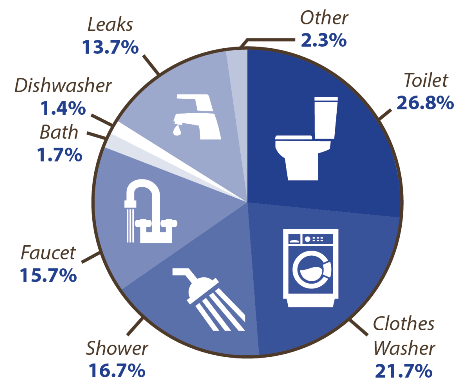# Who Pays for Water Leak?#### Typical Residential Customer Billing

• Water Billing System & saving
• Rate: \$4.074 per 1000 gallon
• Time : 24Hrs
• Total leak (1000 litres * 24) = 24,000/3.7854 = 6340.148 gal
• Cost \$25.829

#### Un-detected water Leak for 1-wk @ 1000 L/hr

• Giving \$4.074 per 1000 gal(Municipal charges)
• Time (1-wk) 7-days (24 * 7) = 168 hr
• Flow rate = 1000L/hr
• Total cost (1000 * 168)/3.785L = 44381 gal
• \$180.808

#### Un-detected Water Leak for 4-wk @ 1000 L/hr

• \$4.074 per 1000 gal
• Time (4-wk) 28-days (24*7*4)= 672
• Flow rate = 1000 L/hr (non eco toilet)
• Total cost (1000*672)/3.785L = 177542.9 gal = \$723.309

#### Un-detected Water Leak for 4-wk @ 1000 L/hr

• \$4.074 per 1000 gal
• Time (4-wk) 28-days (24*7*4)= 672
• Flow rate = 1000 L/hr (non eco toilet)
• Apt building with 400 suites
• Assume 2% leakage (8 suites)
• Total cost (8000*8736)/3.785L=(1420343.46)/(1000)*4.074 gal = \$5,786.46

#### Un-detected Water Leak for 52-wk @ 1000 L/hr

• \$4.074 per 1000 gal
• Time (52-wk) 365-days (24*7*52)= 8736 hrs
• Flow rate = 1000 L/hr (non eco toilet)
• Apt building with 400 suites
• Assume 2% leakage (8 suites) = 8*1000 l/hr
• Total cost (8000*8736)/3.785L=(18464464.99)/(1000)*4.074 gal = \$75,224